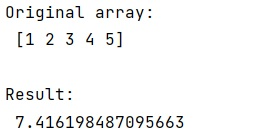# How do you get the magnitude of a vector in NumPy?

Learn, how to get the magnitude of a vector in NumPy?
Submitted by Pranit Sharma, on December 21, 2022

NumPy is an abbreviated form of Numerical Python. It is used for different types of scientific operations in python. Numpy is a vast library in python which is used for almost every kind of scientific or mathematical operation. It is itself an array which is a collection of various methods and functions for processing the arrays.

## Finding the magnitude of a vector in NumPy

According to Linear algebra, an object having both direction and magnitude are known as vectors. Numpy arrays can also be used to depict a vector.

To get the magnitude of a vector in NumPy, we can either define a function that computes the magnitude of a given vector based on a formula or we can use the norm() method in linalg module of NumPy. Here, linalg stands for linear algebra.

The numpy.linalg.norm() method is used to get the magnitude of a vector in NumPy.

We can also pass an optional ord argument for the nth-order norm that we want.

Let us understand with the help of an example,

## Python code to get the magnitude of a vector in NumPy

```# Import numpy
import numpy as np

# Creating a numpy array
arr = np.array([1,2,3,4,5])

# Display original array
print("Original array:\n",arr,"\n")

# Using linalg norm method
res = np.linalg.norm(arr)

# Display Result
print("Result:\n",res)
```

Output: